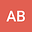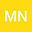Two-phase Stefan problem with nonlinear thermal coefficients and a convective boundary condition
••We consider a non-linear two-phase unidimensional Stefan problem, which consists on a solidification process, for a semi-infinite material $$x>0$$, with phase change temperature $$T_{1},$$ an initial temperature $$T_{2}>T_{1}$$ and a convective boundary condition imposed at the fixed face $$x=0$$ characterized by a heat transfer coefficient $$h>0$$. We assume that the volumetric heat capacity and the thermal conductivity are particular nonlinear functions of the temperature in both solid and liquid phases and they verify a Storm-type relation. A certain inequality on the coefficient $$h$$ is established in order to get an instantaneous phase change process. We determine sufficient conditions on the parameters of the problem in order to prove the existence and uniqueness of a parametric explicit solution for the Stefan problem.